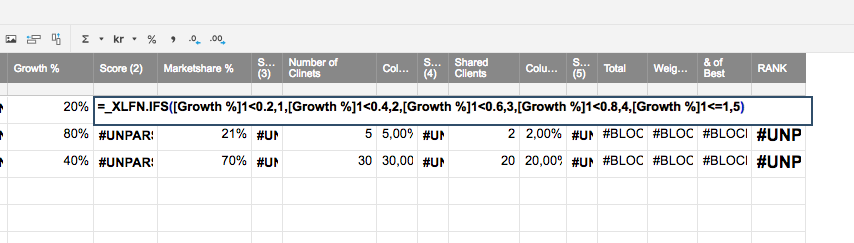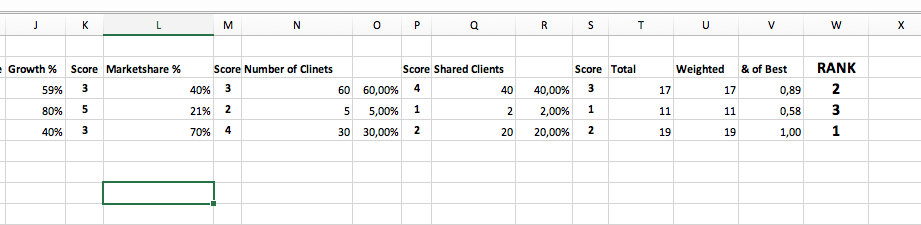# Importing Excel formulas "#UNPARSEABLE"

When trying to import Excel including following formula:

=IFS(J3<0,2;1;J3<0,4;2;J3<0,6;3;J3<0,8;4;J3<=1;5)

I get and error message in Smartsheet. #UNPARSEABLE"

Following show up in Smartsheet.

=_XLFN.IFS([Growth %]1<0.2,1,[Growth %]1<0.4,2,[Growth %]1<0.6,3,[Growth %]1<0.8,4,[Growth %]1<=1,5)

What the formula dose in Excel is to translate percentage to score numbers, Example:

Smaller than 20% will be 1

Smaller than 40% will give 2

Smaller than 60% gives a 3 and so on..

What’s named column J in Excel is equal to the name Growth % in Smartsheet. Many thanks for any recommendations.Tags:

• Hi Roger,

Try this:

=IF([Growth %]1 <= 0,2; 1; IF([Growth %]1 <= 0,4; 2; IF([Growth %]1 <= 0,6; 3; IF([Growth %]1 <= 0,8; 4; IF([Growth %]1 <= 1; 5)))))

Depending on your language you maybe need to change the ; and ,

I hope this helps you!

Best,

Andrée Starå - Workflow Consultant @ Get Done

SMARTSHEET EXPERT CONSULTANT & PARTNER

Andrée Starå | Workflow Consultant / CEO @ WORK BOLD

W: www.workbold.com | E: [email protected] | P: +46 (0) - 72 - 510 99 35

Feel free to contact me about help with Smartsheet, integrations, general workflow advice, or something else entirely.

• When the progress is linear like this, I prefer to use math instead of Nested IF's

=MIN(INT([Growth %]@row * 5) + 1, 5)

But ... I'm weird.

Craig